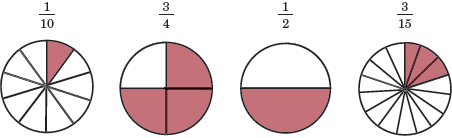# Proper fraction

A proper fraction is a common fraction that represents a quantity less than 1. In other words, the integer in the numerator of the fraction is less than that in the denominator. The figure below depicts 4 diferent proper fractions along with visual representations of the respective fractions.In all of the above fractions, the numerator is smaller than the denominator, making them proper fractions.

A fraction that has a numerator that is greater than or equal to its denominator is referred to as an improper fraction.

Examples

Determine whether the following fractions are proper or improper fractions:1.is a proper fraction because 7 < 8.

2.is an improper fraction because the numerator is equal to the denominator.

3.is an improper fraction because 9 > 8.

## Types of fractions

There are three general forms a fraction: proper fractions, improper fractions, and mixed numbers (or mixed fractions). Improper fractions and mixed numbers are similar in that they both have values greater than or equal to 1. This is in contrast to proper fractions which have a value less than 1.

The difference between improper fractions and mixed numbers is in how they are written. Improper fractions are written as common fractions in which the numerator is greater than or equal to the denominator. On the other hand, mixed numbers are written as a combination of a whole number and a proper fraction.

Below is a figure depicting the 3 different types of fractions.

The figure also shows that both mixed numbers and improper fractions have values greater than 1 while proper fractions have values less than 1. In this specific case, the proper fraction has a value of 0.417 and the mixed number and improper fraction have a value of 2.4.